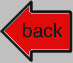Dislocation Science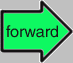1. The Basics

Perfect DislocationsFirst we need to make dislocations in a general and scientific way. It's easy but tends to boggle the mind a bit so I will introduce the procedure bit by bit.
For starters, we need an essential if trivial insight. Let's imagine that we cut a crystal lattice along one of its major planes with an imaginary knife into two parts as shown below: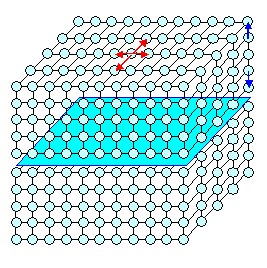1.   First imagine a crystal lattice. Do not confuse it with a crystal. The figure shows a simple cubic lattice because that is easy to draw. 2.   Make a virtual cut through your lattice, e.g. as indicated by the blue plane. The cut could be on any plane; the blue one was chosen because it is simple to draw. 3.   Move the upper part of the lattice relative to the lower part by some lattice translation vector found in the blue plane. This can be any vector obtained by combining integer multiples of the four red base vectors shown. 4.   After the movement has been made, join the two lattices again. First step in making dislocations.The point of the exercise is to make you realize that any movements involving only the red base vectors (including any combination) will retain the perfect fit between the two halves of the lattice. This measn we can join the two halves again without any problems. I won't say more. If you don't get this, look at the pictures below.
Before we look at this in more detail, we need a rule for translations with components perpendicular to the cut plane (involving the blue base vectors). The rule is:
If you do this you must take out or add lattice points / unit cells as required.
Obviously, there are always integer amounts of lattice points or units cells of the lattice that are need to be inserted or must be taken out to generate a smooth lattice once more after joining the two halves.
Here is the illustrations for this: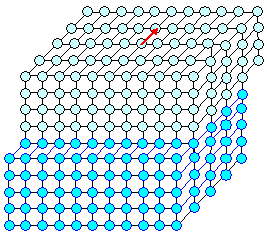Sliding one base vector in the cut plane and joining The upper half was moved back by the red base vector. After joining, the lattice is perfect once more.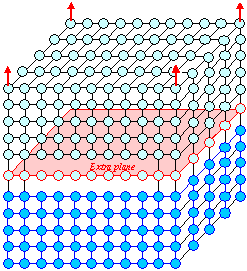Moving up one base vector perpendicular to the cut plane and filling in one additional lattice plane. After the move there is space for exactly one more lattice plane. After filling in that plane and joining, the lattice is perfect again. Same thing for moving in the opposite direction - but difficult to draw. A lattice plane then needs to be taken out; perfect lattice once more after joining.So far it is easy. Now let's make a dislocation in the most general way possible. We only need to modify our game from above a little bit: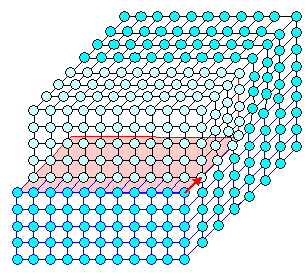1.   Cut into a crystal lattice but do not cut all the way through. 2.   The end of the cut defines a line that runs through the lattice until it emerges at some surface. It cannot end in the lattice. 3.   Move the upper part in the cut area by any base vector (combination) you like, e.g. the red one in the figure. 4.   Since you cannot move beyond the cut line, you adjust the lattice there by squeezing a bit as required and by letting lattice planes end if necessary. Making a dislocation by cutting and moving.We have made an edge dislocation. The cut line defines the dislocation line and the base vector used for the shifting will be what we call the Burgers vector of the dislocation from now on. We just as well could have named it "shove vector" but we honor the work of Johannes Martinus Burgers this way (so it's not Burger's vector!)If you look at the lattice picture above sideways, it looks like this: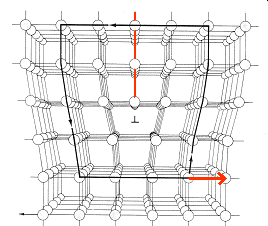Edge dislocation with a Burger's circuitThis is a variant of the "picture" of an edge dislocation that I used in the main text to introduce dislocations. It also shows that just having a picture like that, which may not be just a figure somebody has drawn on paper but a real picture from an electron microscope, allows you to reconstruct the Burgers vector, by doing a so-called Burgers circuit:Just run a loop around the dislocation core (that's why it's called Burgers circuit) that would close in a perfect lattice (e.g. 5 up, 4 left, 5 down, 4 right in the example above). The same kind of loop around a dislocation will not close, and the vector left over (or needed to close the loop) is the Burgers vector of the dislocation. We won't worry about the sign here, i.e. if the Burgers vector points left or right, and that means we don't have to worry about doing the circuit clockwise or counter-clockwise, closed in the perfect lattice or around the dislocation, and so on.
For our edge dislocation the Burgers vector is obviously at right angles to the dislocation line direction. Whenever we find that, we call the dislocation an edge dislocation.So far this hasn't been a big deal. We have "made" edge dislocations in a less abstract way before. However, we are now in a position to make far stranger kinds of dislocations than you ever wanted to know. So let's get to work.
To make things a bit easier, you might imagine the figures that follow to depict a crystal and not a lattice. All you need to do is to imagine that whatever atomic base defines our crystal, is now in place at every point of whatever Bravais lattice we have. In the simplest case, instead of a lattice point you have now one atom. Schematic drawings look the same in both cases. We don't even draw the full lattice anymore. Here are a few simple examples.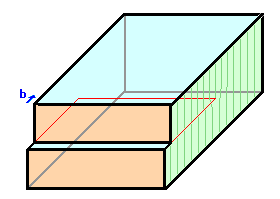Here is the edge dislocation once more, as we know and love it by now. It is just rendered in a more stylized kind of figure. You see the cut line, outlined in red, and the Burgers vector b given in blue. The dislocation line is straight, and it is clear that it can never end inside the crystal.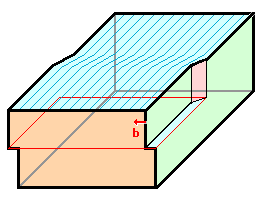Here is a screw dislocation. Same procedure as before, just shove in the direction of the red Burgers vector now. The dislocation line is still straight and it is clear that it can never end inside the crystal. There is no ending lattice plane, however.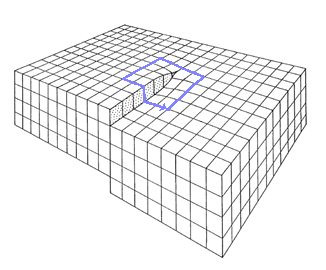Screw dislocation This is just another way of drawing a screw dislocation. It also shows how a Burger's circuit is done. In this case it closes around the dislocation.Screw dislocations have their Bugers vector parallel to the dislocations line. In terms of the atomic configuration, screw dislocations are far trickier to draw than edge dislocations. There is no ending lattice plane and no simple perspective view.
Here is one way of giving some idea of the atomic arrangement in a simple cubic lattice, or if you take the spheres to be atoms, cubic primitive crystal (that does not exist in nature, however).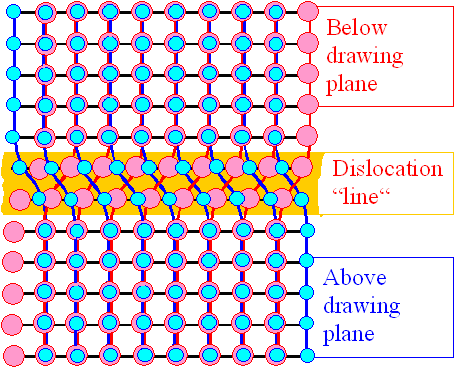Schematic arrangement of atoms in a screw dislocation The cut-plane is the image plane. Shown are the atoms just below (pink) and above (blue) the cut-plane. The arrangement will be symmetrical. Even if you move only the upper plane in your mind, the real crystal will share the movement between the two planes.We are not talking theory here but reality. Here is a high-resolution transmission electron microscope picture of screw dislocations. Shown are the lattice planes above and perpendicular to the "cutting" plane or the plane where the dislocation line resides. It's like looking just at the pink lines in the figure above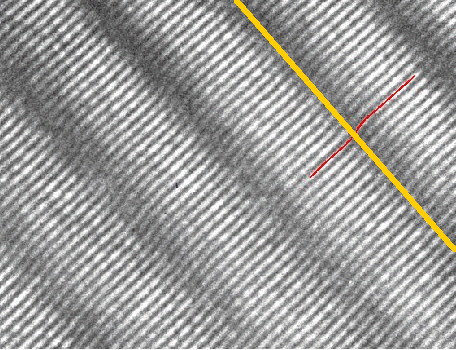Electron microscope picture of screw dislocations Source: H. Föll, D. G. Ast: TEM observations on grain boundaries in sintered Si. Phil. Mag. 40 (1979) 589We see a set of {111} lattice planes above or below (take your own choice) of the "cutting" plane. One of the dislocation lines is indicated in yellow; the red line traces one lattice plane across a dislocation.
This was the very first high-resolution picture of a screw dislocation; it was taken by me around 1979 with the Siemens Elmiskop 102 of the Mat. Science and Eng. Department of Cornell University.We could go on exploring all the possible shifts or Burger vectors that come with one straight cut. We could make many different kinds of perfect dislocations this way. But all we would get as long as we keep the Burgers vector in the plane of the cut, are "mixtures" of edge and screw dislocations with some angle other than 90o or 0o between dislocation line and Burgers vector. So let's be bold and go for another step in generalizing dislocations.
Just realize that there is no need that your cut ends in a straight line. With a virtual knife it is easy to make cuts of any shape. You might, for example, cut in a quarter circle as shown below, and then shift as shown.With a little care and hard thinking, one can still draw a "picture" of what the perfect dislocation produced looks like in terms of the atom arrangement. On the left hand side, it is a screw dislocation, on the right-hand side it is an edge dislocation, in between it is "in between" or mixed dislocation.
We call this and all the other ones made so far a "perfect" dislocation because its Burgers vector is a translation vector of the lattice. A perfect dislocation can exist all by itself in its host lattice, and the crystal around it stays perfect if we don't count a little elastic deformation.
This implies that there are also imperfect dislocations. Yes, there are. Don't worry, we will encounter them soon enough.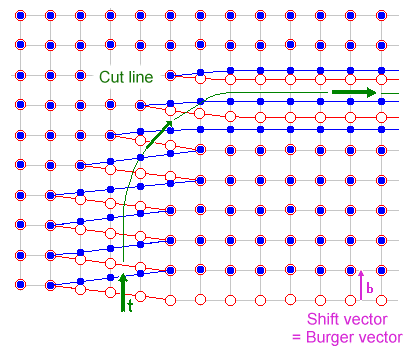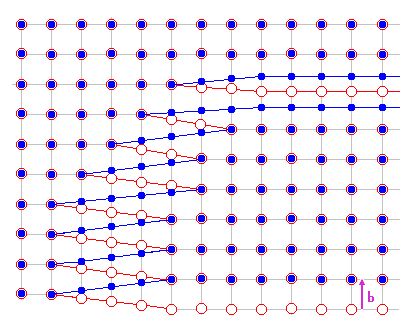Mixed dislocation, changing from "screw" to "edge" type and moving out of the crystal in the lower figure.The figure helps to understand the basic description of a general perfect dislocation:
• It has a line vector named t in the drawing above that describes the "path " of the dislocation through the crystal; i.e. t=t(x, y, z).
• It has a Burgers vector b that is the same everywhere and a translation vector of the lattice.
• It can move on the plane defined by these two vectors. This plane we call "glide plane". This is self-explaining if you look at the animation and imagine that you can move the dislocation by retreating or deepening your cut. As a direct consequence screw dislocations can move on any plane and thus are more mobile than edge dislocations.
• It can be made by making a tiny little "cut" first that is then increased step by step. Just reverse the "moving out" motion in the picture above and you have an illustration of how dislocations nucleate and and "move in" a crystal.It is easy to see that this business of exploring all kinds of cuts and all kinds of shifts gets tiresome after a while. Generating schematic pictures gets more and more involved but there is less and less one can learn from this.
Time to invoke the First law of applied Science. Let's forget about drawing qualitative pictures of all the kinds of dislocations we can think of, and just express the relative displacement of atoms in the vicinity of all dislocations, including the ones we can't think of, by some general equations.In other words, we are now going for the strain or stress tensor associated with a dislocation.
Sorry about that. If you haven't looked at the "science of deformation" module, the time to do that has definitely come.

Stress and Strain Tensor of a DislocationWhile the edge dislocation is easier to conceive than the screw dislocations, it is the other way around if you go for equations. Let's just jump into cold water; explanation will come later. Much of what follows is based on the marvellous book of D. Hull and D.J. Bacon: "Introduction to dislocations".
Here is the strain and stress tensor that "goes" with a screw dislocation that extends in the z-direction of a Cartesian x, y, z coordinate system. It is only an approximation and not valid right at the dislocation core.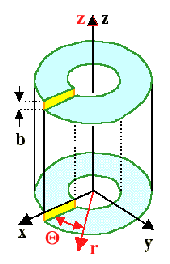e(x, y, z)  = æ ç è ö ÷ ø

exz  =  ezx = –  b
4
· y
x2 + y2
= –  b
4p
· sin Q
r

eyz  =  ezy =   b
4p
· x
x2 + y2
=  b
4p
· cos Q
r

Strain Tensor of a screw dislocation in black Cartesian coordinates (top)
with components given in the (here far easier) red cylinder coordinatesUsing the red cylinder coordinates as shown in the picture seems to make the equations simpler so let's switch to cylidrical coordinates completely. The relations between the two coordinate systems are obvious (r2=x2 + y2; x/r=cosQ, z=z), and we get for strains and stress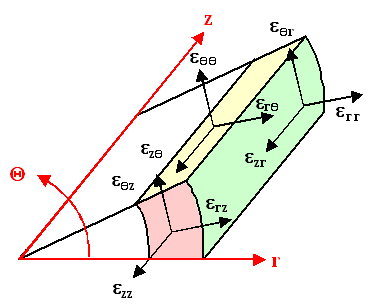eq z  =  ezq  =  b
4pr
sq z   =  szq =  G · b
2pr
Volume element and non-zero components of strain and stress tensor of a screw dislocation in cylinder coordinatesHow does one get the strain tensor? It's actually not all that difficult but I will defer this question to a later module. How does one get the stress tensor if one has the strain tensor? In the equations above its obviously done by multiplying the strain tensor by G/2 (G is the shear modulus) but is that always true?Well - no. The general relation between stress and strain in linear elasticity theory is sij=Sklcijkl · ekl. The elastic coefficients cijkl (a fourth-rank tensor) now replace Young's modulus. You find all that in this module.
Mercifully only two components of cijkl are non-zero for cubic crystals and with luck only one shows up as in the stress expression for the screw dislocation above.Just for completeness, here is the stress tensor for the edge dislocation

sxx   =  – D · y  3x2  +  y2
(x2  +  y2)2

syy   =  D · y  x2  –  y2
(x2  +  y2)2

sxy  =  syx =  D · x x2  –  y2
(x2  +  y2)2

szz  =  n · (sxx + syy)
szz  =  szx  =  yz = szy = 0

D  =  Gb
2p · (1 – n)

G
b
n
=
=
=
Shear modulus
Burges vector
Poisson's ratio
Stress Tensor of an edge dislocationOf course, nobody in her right mind would choose to express stress and strain around a dislocation in a Cartesian coordinate system, when quite obviously a cylinder coordinate system is much better suited to the task. Changing to cylinder coordinates as shown, we get rather simple equations for the two extremes screw and edge dislocation:It's kind of hard to illustrate a tensor. You would need a perspective drawing for each of its components. I can't do that, so here is a standard version showing only some aspects.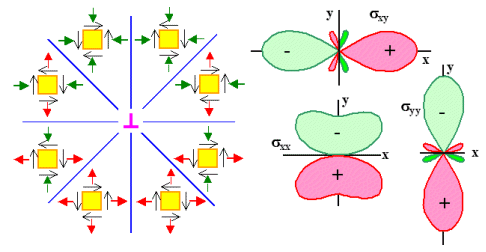Schematic force components on unit cube Lines of constant stress for the three components Stress / strain field around an edge dislocationSince there is no stress perpendicular to the image plane, a two-dimensional representation is sufficient. On the left half of the picture, the forces acting on elementary cubes around the dislocation are shown. They give qualitatively the components of the stress tensor. Green or red denote compressive or tensile normal stresses, respectively.
On the right half, contours of equal stress are shown for the normal component and the shear components of the stress tensor.As far as mixed dislocations are concerned, you simply (haha) combine the equations for the extreme case in a kind of weighted average. What you get covers everything that exists in terms of perfect dislocations.
Just be aware of the fact that the equations above are actually approximations for cubic crystals. They are only valid if you are a bit off from the center of the dislocation (around one or two atomic distances) . At the very core, you need to go to far more complex formulations.Why do I subject you to all those equations? I daresay that very few of you will now be close to an orgasm, hoisting out the champagne and exclaiming: "Isn't that wonderful and exciting! Now I have seen it all and my life finally starts to make sense!"
Well, I did it for the following reasons:
1. In the Science of General Deformation module I I promised that we would "look at some very simple stuff somewhat later". Now I have kept my promise.
2. I wanted to demonstrate that dislocation science is not just about drawing fancy pictures. Those equations are the starting point for to calculating simple and extremely useful quantities. I will do this right below. Then they are also the starting point of calculating everything dislocations do; it's just no longer simple.
3. I'm showing off a bit. This is where the science of hardness (and much else) of steel starts. If you want to make some inroad in this field as a scientist, you do need to apply yourself a bit to the basics first. There are no shortcuts, unfortunatelyBut now let's look at the simple and extremely useful quantities: the energy of a dislocation and the forces between them.

Energy of and Forces between DislocationsWhen you "make" a dislocations you need to shove a lot of atoms around a bit and that takes work or energy. The work invested after all is done is stored in the dislocation, or, to be more precise, in all the bond "springs" that needed to be pushed or pulled a bit relative to their normal length when we make a dislocation.Work equals force times distance. Specific work, i.e. work per unit of length is proportional to stress times strain. For the elastic energy part Eel of the dislocation energy, which is the part outside the core region with a radius ro, we obtain with the equations from above

Eel(screw) R ó õ roDoing the integral takes a bit of fuzzing around with tensor multiplication, integration, and so on, but the result is (almost) easy. The energy depends somewhat on the dimension R of crystal or grain of a poly crystal but we do not need to worry about that.
After doing the same thing for an edge dislocation, coming up with some expression for the energy contained in the core regions (more difficult) one eventually realizes that the energy per unit length (» length of the Burgers vector) of any dislocation is in a fair enough approximation simply given by

Edisl  »  G · b2A really simple formula with a lot of power. It tells us, for example:
1. Dislocations tend to be as short as possible and thus straight lines. That simply minimizes the energy since it scales with the length.
2. The Burgers vector b of a dislocations is always one of the shortest possible translation vectors T0 of the lattice.
If there would be, for example, a dislocation with b=2T0, this dislocations would decompose into two dislocations with b'=T0 each since 2|T0|2 < |2T0|2
3. Two identical dislocations must repel each other if they get close since their strain field and thus their energy adds up. If their Burger vectors have different signs then they must attract each other.With the last point I introduced forces between dislocations. The simple rule is that you add the strain fields as a function of distance. If the combined strain field gets larger as the dislocations get closer, there is a repellent force. If it gets smaller, there is an attractive force.In general equations it tends to get messy so I will not delve into that all that much. The basic situation is shown in the figure below. All dislocations are perpendicular to the viewing plane, inverted T's or circles symbolize edges or screw dislocations, respectively; change in color=change in sign of the Burgers vector.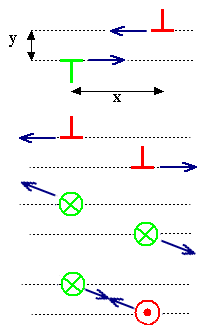Forces on edge dislocations for the geometry shown

Fx  =  Gb2
2p(1 –n)
·  x · (x2y2)
(x2 + y2)2

Fy  =  Gb2
2p(1 –n)
·  y · (3x2 + y2)
(x2 + y2)2
Only the force component in the glide plain (dotted line for the edge dislocations) can move an edge dislocation; this is shown in the figure. A screw dislocation can move or "glide" in any plane that contains it and thus feels the full force.
Forces between dislocations.Of course, we now could also calculate how a dislocation interacts with all kinds of point defects and so on. We won't. It's enough to know that somebody could do it.The next logical step in generalizing our cut-and-shove business would be to cut not in a plane but in whatever irregular shape comes to mind. The cut "plane" then would not be planar and the cut line=dislocation line could be as irregular as a stock market curve.
Be happy that we don't have to deal with that. We go right away for things even worse. We will now consider one parameter we have totally neglected so far: the crystal itself.

Dislocations in CrystalsAll of the above is valid for lattices. You might have imagined atoms or crystals all the time, but they weren't really needed. Essentially, most of what I did so far was an exercise in tricky geometry and the math going with it. In fact, what we now call "dislocations" was already part of a general mathematical treatise from Vito Volterra around 1900, i.e. about 30 years before the "invention" of dislocations in real crystals.So far the the crystal only sneaked in when we looked at energies and forces. Formally that happens whenever in the equations a material property like the shear modulus G comes up.
But just considering energies and forces does not do justice to the full impact a real crystal will have on the properties of dislocationsI won't go into details here but only point out three far-reaching rules that crystals add to the business of dislocations:
1. In lattices, the plane on which the dislocation line can move (the glide plane) is the plane that contains the dislocation line and the Burgers vector. This allows infinitely many combinations. In a crystal, the glide plane is the plane (or the few planes) with the densest packing of atoms. In fcc crystals, for example, this is only the {111} type plane.
2. The Burgers vector is no longer any lattice translation vector but only the shortest possible one. I stated that already above but it is a crystal-induced property.
3. Some of the infinitely many possible directions for the dislocation line have a lower energy than others. In fcc crystals, for example, the <110> direction is the preferred direction and that's where the dislocation tends to extend itself.Did you notice what that means for the cut-and-shove procedure? Let's give it a look:
1. Cuts are now restricted to planes with the largest (or at least a large) packing density of atoms. In fcc crystal that leaves only the 4 sets of {111} type planes (look right).
2. Burgers vectors are restricted to the shortest possible translation vectors of the lattice. In fcc crystals that leaves only the six variants of a/2<110> vectors.
If the figure isn't clear enough, maybe it's time to look at this module?
3. The cut line should be along the "easy" directions of the crystal. In fcc crystals that would be <110>.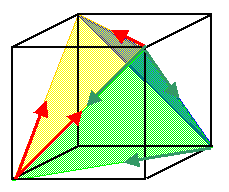Four {111} planes with 3 Burgers vectors each=12 glide systems Illustrat. Module Glide systemsThere is now a limited number of so-called glide systems, meaning combinations of a glide plane (=densely packed plane) and a Burger vector (=shortest possible lattice translation vector). That is of prime importance because the more glide systems you have, the easier it is to deform the crystal.
The illustration module shows this for the three prominent lattice types.Of course, if you belong to the dislocation liberation movement, you will not heed these offending rules but make your cut as a you like. You can do that, no problem. All that will happen is:
• Your dislocations will split into as many dislocation as are needed to provide each one with the desired smallest Burgers vector. Those dislocations will repel each other if you look at the forces between them.
• After splitting, the dislocations will move on their glide planes until they assume the best geometrical arrangement.The second statement is a bit vague. Why didn't I just say: "...until the extend in the favorite direction"? Because I sneaked in a puzzle. We actually now have two mutually conflicting conditions for the direction of dislocation line:
1. The dislocation line between A and B should be as short as possible.
2. The dislocation line between A and B should be in a certain direction.
Just as you cannot simply go from A to B on the shortest possible route and only going true north in almost all cases, the dislocation can't either. The points A and B are the points where the dislocation ends on some surface or interface, or nodes where several dislocations (almost always just three) meet.Both rules are not absolute but just "recommendations". While in electron microscope pictures dislocations tend to be rather straight, there are plenty of examples where they are curved, witness the two examples in the backbone.Well, this is getting complicated. And we are still rather looking at the appetizer and not yet eating it.If you are ready for a full dinner now, order the full menu known as "The Bible":
J.P. Hirth and J. Lothe, Theory of Dislocations, second edition (Krieger Publishing, Malabar Florida 1982).If you are content with just looking at appetizers, you might continue with this module.Back to Dislocation ScienceOn to1. The Basics2. The reality.3. SpecialitiesBooks and Other Major SourcesDefect Etching in SiliconMyths and Bullshit Around QuenchingBravais Lattices and CrystalsSpring Model and Properties of CrystalsInhomogeneous DeformationHeroes of Dislocation ScienceGrain Boundary - AdvancedDislocation ScienceTransmission Electron MicroscopesTwinning, Shear Deformation and Martensite FormationDisplacement and StrainThermal StressMicroscopes for ScienceScience of DeformationHomogeneous NucleationDislocation Science - 2. The RealityDislocation Science - 3. SpecialitiesDeformation Types

© H. Föll (Iron, Steel and Swords script)